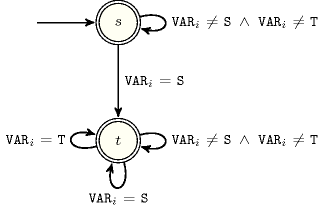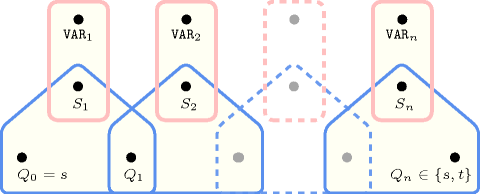## 5.195. int_value_precede

Origin
Constraint

$\mathrm{𝚒𝚗𝚝}_\mathrm{𝚟𝚊𝚕𝚞𝚎}_\mathrm{𝚙𝚛𝚎𝚌𝚎𝚍𝚎}\left(𝚂,𝚃,\mathrm{𝚅𝙰𝚁𝙸𝙰𝙱𝙻𝙴𝚂}\right)$

Synonyms

$\mathrm{𝚙𝚛𝚎𝚌𝚎𝚍𝚎}$, $\mathrm{𝚙𝚛𝚎𝚌𝚎𝚍𝚎𝚗𝚌𝚎}$, $\mathrm{𝚟𝚊𝚕𝚞𝚎}_\mathrm{𝚙𝚛𝚎𝚌𝚎𝚍𝚎}$.

Arguments
 $𝚂$ $\mathrm{𝚒𝚗𝚝}$ $𝚃$ $\mathrm{𝚒𝚗𝚝}$ $\mathrm{𝚅𝙰𝚁𝙸𝙰𝙱𝙻𝙴𝚂}$ $\mathrm{𝚌𝚘𝚕𝚕𝚎𝚌𝚝𝚒𝚘𝚗}\left(\mathrm{𝚟𝚊𝚛}-\mathrm{𝚍𝚟𝚊𝚛}\right)$
Restrictions
 $𝚂\ne 𝚃$ $\mathrm{𝚛𝚎𝚚𝚞𝚒𝚛𝚎𝚍}$$\left(\mathrm{𝚅𝙰𝚁𝙸𝙰𝙱𝙻𝙴𝚂},\mathrm{𝚟𝚊𝚛}\right)$
Purpose

If value $𝚃$ occurs in the collection of variables $\mathrm{𝚅𝙰𝚁𝙸𝙰𝙱𝙻𝙴𝚂}$ then its first occurrence should be preceded by an occurrence of value $𝚂$.

Example
$\left(0,1,〈4,0,6,1,0〉\right)$

The $\mathrm{𝚒𝚗𝚝}_\mathrm{𝚟𝚊𝚕𝚞𝚎}_\mathrm{𝚙𝚛𝚎𝚌𝚎𝚍𝚎}$ constraint holds since the first occurrence of value 0 precedes the first occurrence of value 1.

Typical
 $𝚂<𝚃$ $|\mathrm{𝚅𝙰𝚁𝙸𝙰𝙱𝙻𝙴𝚂}|>1$ $\mathrm{𝚊𝚝𝚕𝚎𝚊𝚜𝚝}$$\left(1,\mathrm{𝚅𝙰𝚁𝙸𝙰𝙱𝙻𝙴𝚂},𝚂\right)$ $\mathrm{𝚊𝚝𝚕𝚎𝚊𝚜𝚝}$$\left(1,\mathrm{𝚅𝙰𝚁𝙸𝙰𝙱𝙻𝙴𝚂},𝚃\right)$
Symmetries
• An occurrence of a value of $\mathrm{𝚅𝙰𝚁𝙸𝙰𝙱𝙻𝙴𝚂}.\mathrm{𝚟𝚊𝚛}$ that is different from $𝚂$ and $𝚃$ can be replaced by any other value that is also different from $𝚂$ and $𝚃$.

• All occurrences of values $𝚂$ and $𝚃$ can be swapped in $𝚂$, $𝚃$ and $\mathrm{𝚅𝙰𝚁𝙸𝙰𝙱𝙻𝙴𝚂}.\mathrm{𝚟𝚊𝚛}$.

Arg. properties
• Suffix-contractible wrt. $\mathrm{𝚅𝙰𝚁𝙸𝙰𝙱𝙻𝙴𝚂}$.

• Aggregate: $𝚂\left(\mathrm{𝚒𝚍}\right)$, $𝚃\left(\mathrm{𝚒𝚍}\right)$, $\mathrm{𝚅𝙰𝚁𝙸𝙰𝙱𝙻𝙴𝚂}\left(\mathrm{𝚞𝚗𝚒𝚘𝚗}\right)$.

Algorithm

A filtering algorithm for maintaining value precedence is presented in [YatChiuLawJimmyLee04]. Its complexity is linear to the number of variables of the collection $\mathrm{𝚅𝙰𝚁𝙸𝙰𝙱𝙻𝙴𝚂}$.

Systems
generalisation: $\mathrm{𝚒𝚗𝚝}_\mathrm{𝚟𝚊𝚕𝚞𝚎}_\mathrm{𝚙𝚛𝚎𝚌𝚎𝚍𝚎}_\mathrm{𝚌𝚑𝚊𝚒𝚗}$ ($\mathrm{𝚜𝚎𝚚𝚞𝚎𝚗𝚌𝚎}$ of 2 $\mathrm{𝚟𝚊𝚕𝚞𝚎𝚜}$ replaced by $\mathrm{𝚜𝚎𝚚𝚞𝚎𝚗𝚌𝚎}$ of at least 2 $\mathrm{𝚟𝚊𝚕𝚞𝚎𝚜}$), $\mathrm{𝚜𝚎𝚝}_\mathrm{𝚟𝚊𝚕𝚞𝚎}_\mathrm{𝚙𝚛𝚎𝚌𝚎𝚍𝚎}$ ($\mathrm{𝚜𝚎𝚚𝚞𝚎𝚗𝚌𝚎}$ of $\mathrm{𝚍𝚘𝚖𝚊𝚒𝚗}\mathrm{𝚟𝚊𝚛𝚒𝚊𝚋𝚕𝚎𝚜}$ replaced by $\mathrm{𝚜𝚎𝚚𝚞𝚎𝚗𝚌𝚎}$ of $\mathrm{𝚜𝚎𝚝}$ $\mathrm{𝚟𝚊𝚛𝚒𝚊𝚋𝚕𝚎𝚜}$).
Figure 5.195.1 depicts the automaton associated with the $\mathrm{𝚒𝚗𝚝}_\mathrm{𝚟𝚊𝚕𝚞𝚎}_\mathrm{𝚙𝚛𝚎𝚌𝚎𝚍𝚎}$ constraint. Let ${\mathrm{𝚅𝙰𝚁}}_{i}$ be the ${i}^{th}$ variable of the $\mathrm{𝚅𝙰𝚁𝙸𝙰𝙱𝙻𝙴𝚂}$ collection. To each triple $\left(𝚂,𝚃,{\mathrm{𝚅𝙰𝚁}}_{i}\right)$ corresponds a signature variable ${S}_{i}$ as well as the following signature constraint: $\left({\mathrm{𝚅𝙰𝚁}}_{i}=𝚂⇔{S}_{i}=1\right)\wedge \left({\mathrm{𝚅𝙰𝚁}}_{i}=𝚃⇔{S}_{i}=2\right)\wedge \left({\mathrm{𝚅𝙰𝚁}}_{i}\ne 𝚂\wedge {\mathrm{𝚅𝙰𝚁}}_{i}\ne 𝚃⇔{S}_{i}=3\right)$.
##### Figure 5.195.1. Automaton of the $\mathrm{𝚒𝚗𝚝}_\mathrm{𝚟𝚊𝚕𝚞𝚎}_\mathrm{𝚙𝚛𝚎𝚌𝚎𝚍𝚎}$ constraint (state $s$ means that value $𝚂$ was not yet encountered, while state $t$ means that value $𝚂$ was already encountered)##### Figure 5.195.2. Hypergraph of the reformulation corresponding to the automaton of the $\mathrm{𝚒𝚗𝚝}_\mathrm{𝚟𝚊𝚕𝚞𝚎}_\mathrm{𝚙𝚛𝚎𝚌𝚎𝚍𝚎}$ constraint